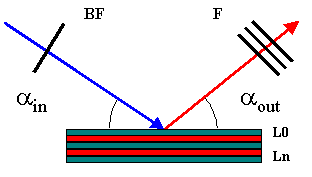Application to calculate, using the fisx library, the expected x-ray intensities of an element given a sample, geometry and detection system. At the very minimum the application needs the complete description of one sample layer (material, density and thickness) and the list of elements and lines to be evaluated. You can define your own materials either by providing a formula or by providing a name and then defining its composition. Calculation is performed by pressing "Submit" at the bottom of this page. Output gives the enhancement due to Secondary excitation and to Secondary plus Tertiary excitation as well as the expected rate per unit mass fraction of the element in the sample. The actual rate is just the multiplication of the previous rate by the mass fraction of the element in the sample. If a detector is supplied, the escape peak intensities and solid angle will be considered in the calculation.Excitation Beam
keV
Geometry
deg.
deg.
Materials
Matrix Multilayer definition
Material Density (g/cm 3 ) Thickness (cm)
Beam Filters
Material Density (g/cm 3 ) Thickness (cm) Funny factor
Filters
Material Density (g/cm 3 ) Thickness (cm) Funny factor
Detector
Material Density (g/cm 3 ) Thickness (cm) Detector area (cm 2 ) Distance (cm)
Peaks
• H Hydrogen
• He Helium
• Li Lithium
• Be Beryllium
• B Boron
• C Carbon
• N Nitrogen
• O Oxygen
• F Fluorine
• Ne Neon
• Na Sodium
• Mg Magnesium
• Al Aluminium
• Si Silicon
• P Phosphorus
• S Sulfur
• Cl Chlorine
• Ar Argon
• K Potassium
• Ca Calcium
• Sc Scandium
• Ti Titanium
• Cr Chromium
• Mn Manganese
• Fe Iron
• Co Cobalt
• Ni Nickel
• Cu Copper
• Zn Zinc
• Ga Gallium
• Ge Germanium
• As Arsenic
• Se Selenium
• Br Bromine
• Kr Krypton
• Rb Rubidium
• Sr Strontium
• Y Yttrium
• Zr Zirconium
• Nb Niobium
• Mo Molybdenum
• Tc Technetium
• Ru Ruthenium
• Rh Rhodium
• Ag Silver
• In Indium
• Sn Tin
• Sb Antimony
• Te Tellurium
• I Iodine
• Xe Xenon
• Cs Caesium
• Ba Barium
• La-Lu Lanthanide
• Hf Hafnium
• Ta Tantalum
• W Tungsten
• Re Rhenium
• Os Osmium
• Ir Iridium
• Pt Platinum
• Au Gold
• Hg Mercury
• Tl Thallium
• Bi Bismuth
• Po Polonium
• At Astatine
• Fr Francium
• Ac-Lr Actinide
• Rf Rutherfodum
• Db Dubnium
• Sg Seaborgium
• Bh Bohrium
• Hs Hassium
• Mt Meitnerium
• Rg Roentgenium
• Uub Ununbium
• Uut Ununtrium
• Uup Ununpentium
• Uuh Ununhexium
• Uus Ununseptum
• Uuo Ununoctium
• C Solid
• Hg Liquid
• H Gas
• Rf Unknown
`   `